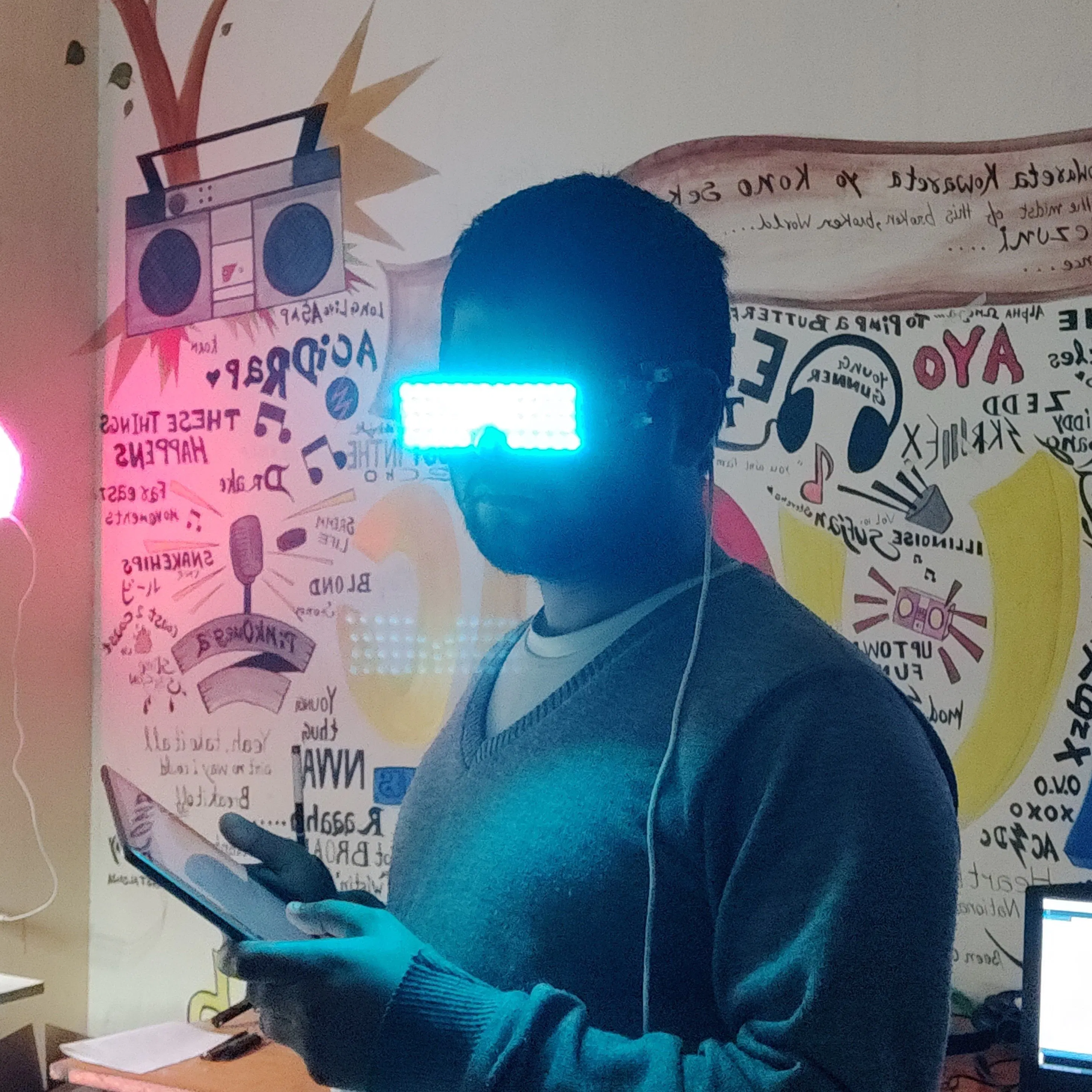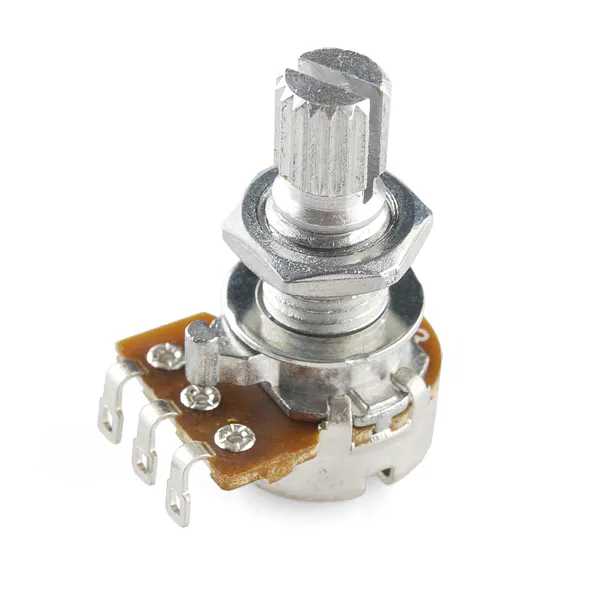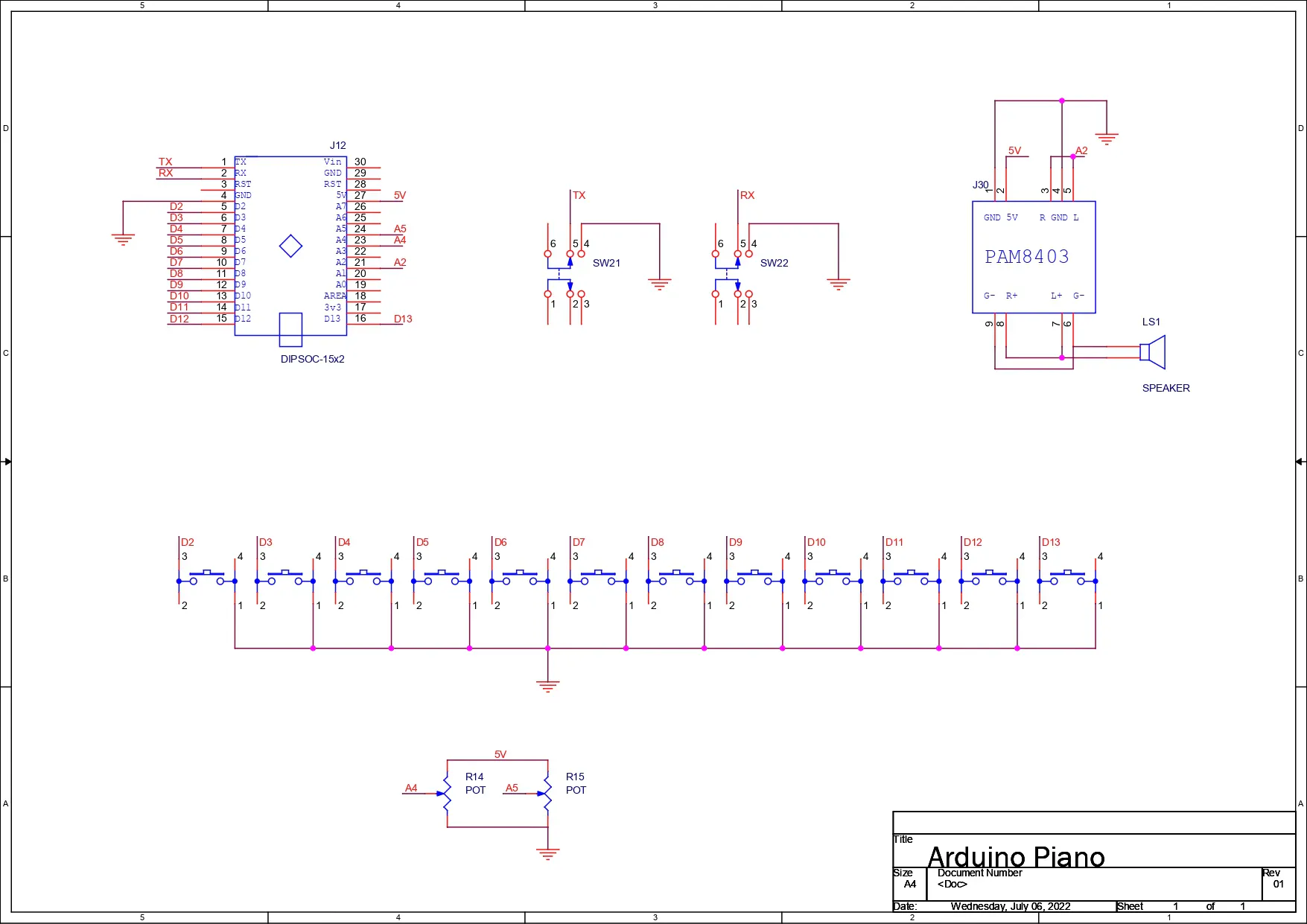# Arduino Synth V3

A simple Arduino-based synth that can generate all sorts of exciting sounds.

BeginnerFull instructions provided1 hour175

## Things used in this project

### Hardware componentsPCBWay Custom PCB
×1Arduino Nano R3
×1SparkFun Pushbutton switch 12mm
×12Rotary potentiometer (generic)
×2
 PAM8403 Module
×1

### Software apps and online servicesArduino IDE

## Schematics

### sch## Code

### code

C/C++
```int C  = 2;
int CS = 3;
int D  = 4;
int DS = 5;
int E  = 6;
int F  = 7;
int FS = 8;
int G  = 9;
int GS = 10;
int A  = 11;
int AS = 12;
int B  = 13;
int octabajo = 0;
int octarriba = 1;

int c  = 262;
int cs = 277;
int d  = 294;
int ds = 311;
int e  = 330;
int f  = 349;
int fs = 370;
int g  = 392;
int gs = 415;
int a  = 440;
int as = 466;
int b  = 494;

void setup() {
// INPUT CONFIG
pinMode(C, INPUT_PULLUP);
pinMode(CS, INPUT_PULLUP);
pinMode(D, INPUT_PULLUP);
pinMode(DS, INPUT_PULLUP);
pinMode(E, INPUT_PULLUP);
pinMode(F, INPUT_PULLUP);
pinMode(FS, INPUT_PULLUP);
pinMode(G, INPUT_PULLUP);
pinMode(GS, INPUT_PULLUP);
pinMode(A, INPUT_PULLUP);
pinMode(AS, INPUT_PULLUP);
pinMode(B, INPUT_PULLUP);
pinMode(octabajo, INPUT_PULLUP);
pinMode(octarriba, INPUT_PULLUP);
pinMode(A4, INPUT_PULLUP);
// OUTPUT CONFIG
pinMode(A2, OUTPUT);
// start serial port
Serial.begin(9600);
}

void loop() {

// put your main code here, to run repeatedly:

}
}
}

}
}
}

}
}
}

}
}
}

}
}
}

}
}

}
}
}

}
}
}

}
}
}

}
}
}

}
}
}

}
}
}

noTone(A2);

}
```

## Credits

### Arnov Sharma

147 projects • 203 followers